Home > MC2 > Chapter 11 > Lesson 11.2.4 > Problem11-66

11-66.
1. Imagine spinning the spinner below. Homework Help ✎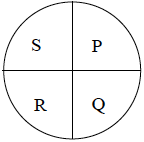1. What is the probability you will spin a P or a Q on your first spin?

2. Find all of the possible outcomes of spinning the spinner twice. What is the probability you will spin a P on your first spin and then a Q on your second spin? Explain how you decided.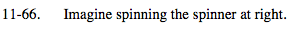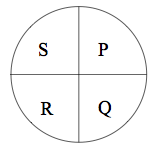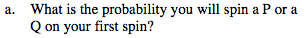How many sections have either a P or a Q?
How many total sections are there?

$\frac{2}{4} = \frac{1}{2}$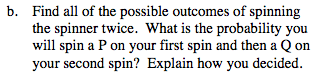Make a tree diagram or a systematic list.
For help refer to the Math Notes box of Lesson 11.2.3.

Make a systematic list by placing the letter that is landed on first
in the front and the letter landed on second next.

SS SP SQ SR
PS PP PQ PR
QS QP QQ QR
RS RP RQ RR

How many possible outcomes are there?

Which symbol represents the outcome of landing on
P first and Q second?